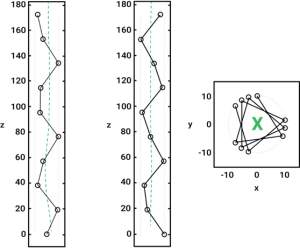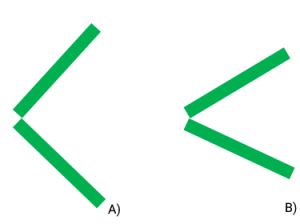Measurand’s Cyclical software adjustment is designed to be used with our SAAV model ShapeArrays. Due to the small diameter of the SAAV’s segments, the instrument will take on a helical, or “zig zagged”, shape when installed in conduits with internal diameters from 27 mm to 100 mm.Figure 1: SAAV’s helically installed shape – dotted green line and X represent the medial axis

The helical installed shape of the SAAV inside the casing can lead to saw-toothed-shaped artifacts in the unadjusted deformation profile. While this profile appears jagged, the movement of each of the vertices in the ShapeArray are accurately represented within a 0.5 mm resolution per segment. To help smooth out the deformation profile, Measurand has developed the cyclical software adjustment. The cyclical software adjustment calculates a medial axis (centreline) through the SAAV’s helical shape and uses changes in the medial axis to calculate the deformation profile. The cyclical software adjustment calculates the medial axis using a two-step process.

The first step in the medial axis calculation is a settlement analysis to identify and remove sudden deformations that can be caused by insufficient compression at the time of installation. As illustrated in Figure 2, the minor localized movements that can be reported by the instrument are linked to small, equal reductions in tilt of adjacent segments in opposite directions. Deformations resulting from this sort of settlement do not represent real soil movement and should be removedFigure 2: An example of the reduction in the angle between adjacent segments due to settlement – A) installed shape, B) after settlement

The second step in the medial axis calculation applies spatial filtering to the ShapeArray’s helical shape to reduce it to a straighter path that runs approximately through the center of the casing.  This is done by averaging the values of pairs of adjacent vertices throughout the SAAV to calculate new values for the vertices. In order to include the top and bottom vertices in the filtering, theoretical vertices are calculated above and below the top and bottom vertices to extend the cyclical shape for the calculation.Figure 3: Virtual vertices are added as part of the spatial filtering process.

Once the virtual vertices have been calculated, each adjacent pair of vertices are then averaged together to produce a new set of vertices. This entire process is then repeated with the newly calculated vertices three times to produce the vertices that define the medial axis. This medial axis is then used in all subsequent deformation calculations.Figure 4: To find the medial axis, new vertices are calculated by averaging pairs of adjacent vertices